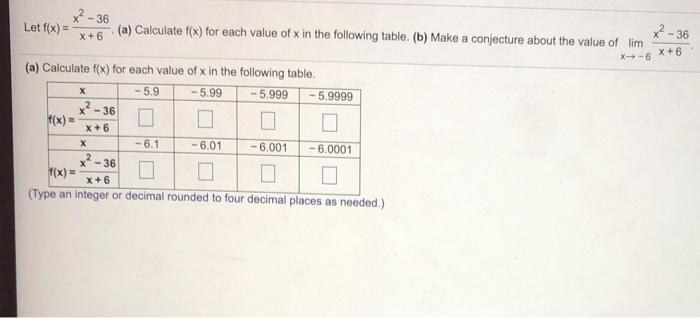### Create an Account

Already have account?

### Forgot Your Password ?

Home / Questions / x2 - 36 Let f(x) = X+ 6 (a) Calculate f(x) for each value of x in the following table. (b)...

# x2 - 36 Let f(x) = X+ 6 (a) Calculate f(x) for each value of x in the following table. (b) Make a conjecture about the value of lim x2 - 36 X-6 X + 6 х f(x) = (a) Calculate f(x) for each value of x in

x2 - 36 Let f(x) = X+ 6 (a) Calculate f(x) for each value of x in the following table. (b) Make a conjecture about the value of lim x2 - 36 X-6 X + 6 х f(x) = (a) Calculate f(x) for each value of x in the following table, -5.9 -5.99 -5.999 5.9999 -36 X+6 -6.1 -6.01 - 6.001 -6.0001 x? - - 36 f(x) = X+6 (Type an integer or decimal rounded to four decimal places as needed.) XApr 15 2021 View more View Less

#### Answer (Solved)Subscribe To Get Solution